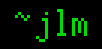## 21-Oct-2014

### Why was algebra so difficult to discover?

Filed under: math — jlm @ 18:48

(tl;dr: Asking a bunch of questions, and not making any guesses.)
Consider integer arithmetic prior to the discovery of algebra. How do you go about a really foundational task such as defining division? You give everything involved names, like so: A number called the dividend divided by another number called the divisor is a number called the quotient with a non-negative number called the remainder if the divisor times the quotient plus the remainder is the dividend and the remainder is smaller than the divisor. How do you get anything done with that kind of word salad? No, when you want to actually do some number theory, you go with: n÷d = q R r is equivalent to n = q∙d + r ∧ 0 ≤ r < d. This terminology is taught because you teach division well before you teach algebra, but it’s so useless afterwards that I very well might have never used this sense of the word “dividend” all these years since I learned algebra. Anyone skeptical that basic grade school arithmetic isn’t far harder to grasp without algebra is invited to explain how come long division gets the right answer without using it.

So fine, algebra is incredibly useful, but utility has no relevance to ease of understanding. I remember having some confusion when I was introduced to it. It was along the lines of: “What number is x?” “That can be any number.” “*boggle*”. Yet, those named terms above, they’re just placeholders too: “What number is the dividend?” “That can be any number.” “Oh, okay.” — How come that’s different?

The Greeks were using letters to represent arbitrary points in geometry centuries earlier than the Arabs did that for arbitrary numbers. In teaching geometry we don’t get the problem “What point is A?” “That can be any point.” “*boggle*” — What makes “x can be any number” so much harder than “A can be any point”?